# Hands

The clock shows 12 hours. After how many minutes will angle between the hour and minute hand 90°?

Consider the continuous movement of both hands hours.

x =  16.36 min

### Step-by-step explanation:

360/60•x - 360/(12•60)•x = 90

330x = 5400

x = 180/11 ≈ 16.363636

Our simple equation calculator calculates it.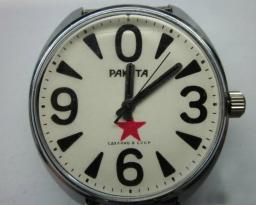Did you find an error or inaccuracy? Feel free to write us. Thank you!Tips to related online calculators
Need help to calculate sum, simplify or multiply fractions? Try our fraction calculator.
Do you have a linear equation or system of equations and looking for its solution? Or do you have a quadratic equation?
Do you want to convert length units?
Do you want to convert velocity (speed) units?
Do you want to convert time units like minutes to seconds?

#### You need to know the following knowledge to solve this word math problem:

We encourage you to watch this tutorial video on this math problem:

## Related math problems and questions:

• Clock hands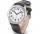The hands-on clock shows the time 12 hours and 2 minutes. Calculate the size of an acute angle between clock hands three hours later.
• Special watchFero bought a special watch on the market. They have only one (minute) hand and a display that shows which angle between the hour and minute hand. How many hours was his watch shown - the minute hand points to number 2; the display shows 125°?
• Minute-hand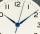How long distance will travel a large (minute) hand on the clock for 48 minutes, if its length is 56 cm?
• Minute handWhat is the distance the minute hand of the clock travels in 12 minutes, if the diameter of the clock is 30 cm and the hand extends to a distance of 2 cm from the edge of the clock?
• Clocks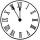What distance will describe the tip of minute hand 6 cm long for 20 minutes when we know the starting position with finally enclose hands each other 120°?
• Clock Tower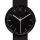What angle is betwenn hands on Clock Tower when show 17 hours and 35 minutes?
• ClocksHow will long path walk the end of the clock's minute-hand long 7 cm for 330 minutes? How long will walk in the same time hour-hand?
• Time clockWhat are the angle of the hour hand and the minute hand if it is 0:40?
• Little hand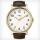What angle shifted little hand on the clock after one hour and 38 minutes?
• Minute hand v2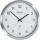In how many minutes describe the minute hand angle 60 degrees?
• Speed of clockIn how many minutes will the hour hand pass a straight angle and a right angle?
• TrianglesFind out whether given sizes of the angles can be interior angles of a triangle: a) 23°10',84°30',72°20' b) 90°,41°33',48°37' c) 14°51',90°,75°49' d) 58°58',59°59',60°3'
• ClockHow many times a day hands on a clock overlap?
• The big clock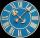The big clock hands stopped at a random moment. What is the probability that: a) a small hand showed the time between 1:00 and 3:00? b) the big hand was in the same area as a small hand in the role of a)? c) did the hours just show the time between 21:00
• Clock handsThe second hand has a length of 1.5 cm. How long does the endpoint of this hand travel in one day?
• LunchLunch is given to seniors from 12:15 to 12:40 during the Coronavirus pandemic. What angle will the minute hand of clock describe during this time?
• Clock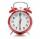What distance will pass end of 8 cm long hour hand for 15 minutes?